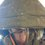# Ω calculation function idea

So at school I was thinking "why don't I create a function that someone can use to calculate numbers in their head" so I came up with the Ω function for calculation, it's basically 1Ω=1..100, 2Ω=2...200 and so on, but how do we get that number? Do we use the Riemann zeta function? Euler gamma function? I need your help to design a concept, comment ideas below!Note by Garrett O’Brien
5 years, 3 months ago

This discussion board is a place to discuss our Daily Challenges and the math and science related to those challenges. Explanations are more than just a solution — they should explain the steps and thinking strategies that you used to obtain the solution. Comments should further the discussion of math and science.

When posting on Brilliant:

• Use the emojis to react to an explanation, whether you're congratulating a job well done , or just really confused .
• Ask specific questions about the challenge or the steps in somebody's explanation. Well-posed questions can add a lot to the discussion, but posting "I don't understand!" doesn't help anyone.
• Try to contribute something new to the discussion, whether it is an extension, generalization or other idea related to the challenge.

MarkdownAppears as
*italics* or _italics_ italics
**bold** or __bold__ bold
- bulleted- list
• bulleted
• list
1. numbered2. list
1. numbered
2. list
Note: you must add a full line of space before and after lists for them to show up correctly
paragraph 1paragraph 2

paragraph 1

paragraph 2

[example link](https://brilliant.org)example link
> This is a quote
This is a quote
    # I indented these lines
# 4 spaces, and now they show
# up as a code block.

print "hello world"
# I indented these lines
# 4 spaces, and now they show
# up as a code block.

print "hello world"
MathAppears as
Remember to wrap math in $$ ... $$ or $ ... $ to ensure proper formatting.
2 \times 3 $2 \times 3$
2^{34} $2^{34}$
a_{i-1} $a_{i-1}$
\frac{2}{3} $\frac{2}{3}$
\sqrt{2} $\sqrt{2}$
\sum_{i=1}^3 $\sum_{i=1}^3$
\sin \theta $\sin \theta$
\boxed{123} $\boxed{123}$

Sort by:

teal u wort, wee carn oose ze raiman zeti fancshion 2 ween at live.

loork at dis:

$\sum _{n=1}^{inf}H_n^2x^n=\frac{1}{x}\left[\sum _{n=1}^{inf}H_n^2x^n+\sum _{n=2}^{inf}\frac{1}{n^2}x^n-2\sum _{n=2}^{inf}\frac{H_n}{n}x^n\right]-1$

$2\sum _{n=1}^{inf}\left(\sum _{k=1}^n\frac{1}{k}\right)^2\left(\frac{1}{2}\right)^n+2L-4\sum _{n=1}^{inf}\frac{\sum _{k=1}^n\frac{1}{k}}{n}\left(\frac{1}{2}\right)^n$

$S=\frac{\pi ^2}{6}+\ln ^2\left(2\right)$

eat's ass eencormprihancibel ass ur stuupeedeeti ees 2 u.

- 5 years, 3 months ago

btw, is there any chance that you might be known as Noted Scholar in other accounts? I'm a big fan of noted scholar.

- 5 years, 3 months ago

You can even write in integral representation:

$\huge n\Omega=\dfrac{ \int_{0}^{\infty} \dfrac{x^{100n}}{\zeta(100n+1)(e^x-1)} \ dx}{ \int_{0}^{\infty} \dfrac{x^n}{\zeta(n+1)(e^x-1)} \ dx}$

- 5 years, 3 months ago

@Ark3 Graptor there?

- 5 years, 3 months ago

- 5 years, 3 months ago

That's quite a bit of detail

- 5 years, 3 months ago

So basically you are defining $n\Omega=\dfrac{(100n)!}{n!}$ right?

- 5 years, 3 months ago

I guess so

- 5 years, 3 months ago

No wait it's this: $1\Omega = 1,2,3,4,5,6,7...100, 2\Omega = 2,4,6,8,10,12,14...200, etc$ The thing is is how to obtain one specific number from that selection withought randomly picking one

- 5 years, 3 months ago

So we have $n\Omega=\dfrac{\Gamma(100n+1)}{\Gamma(n+1)}$ , got till here?

- 5 years, 3 months ago

Better

- 5 years, 3 months ago